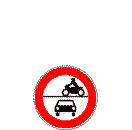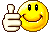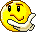## ---> HP4 -eure Seriennummer <---

Das spezielle Forum für/rund um die Besonderheiten zur S 1000 RR - HP 4 - HP4 Race.

### Re: ---> HP4 -eure Seriennummer <---

ich hab 4329Black_out117

Beiträge: 12
Registriert: 20.07.2016, 09:27
Wohnort: Mindelheim

### Re: ---> HP4 -eure Seriennummer <---

0004 = Hans Peter (Competition)
0007 = Griz89 (Competition)
0014 = jleserer (Competition)
0024 = Boogeyman (Competition)
0028 = Rico53 (Competition)
0031 = Nikko
0035 = HpVier
0041 = n U k E (Competition)
0061 = twiti111
0117 = HESI
0131 = S1kRr (Competition)
0149 = emil
0168 = kuehli
0169 = Matthias K (Competition)
0175 = Wolfgang
0183 = kein Forumsmitglied (Competition)
0193 = s_endl (Competition)
0259 = Domino100
0260 = Harald
0266 = kein Forumsmitglied
0270 = Peter [kein Forumsmitglied]
0274 = ZX10r (Competition)
0277 = Toto277
0427 = Twix_72 (Competition) 1.joee014
0500 = FirefoxR1 (Competition)
0657 = Slowbiker
0773 = Langer
0831 = WolfgangBZ
0841 = wilkup
0872 = J.K.
0874 = Cactus
0877 = Steine (Competition)
0879 = M-Power (Competition)
0903 = Peter 64
0904 = mehrschbass
0911 = Stratos-Schorsch (Competition)
0915 = celta, 1.Xurali
0956 = taunus hp
0963 = chaxtec (Competition)
0995 = HROyal
1022 = Remo (Competition)
1023 = freilin8
1044 = Ecotec
1045 = F-RR-anzl
1072 = RRMarkus
1105 = Ralle (Competition)
1117 = Rudolf
1122 = Frankenraudi (Competition)
1134 = Stanley
1190 = Herrenlos (Competition)
1247 = romo (Competition)
1267 = Happe4
1513 = Wastl
1560 = loewenherz
1669 = RS_Dom
1671 = Host-82 (Competition)
1715 = # 66
1716 = BMWerner
1724 = Alkor (Competition)
1730 = EM KA (Competition) [Ex Forumsmitglied]
1753 = Cool60
1761 = SAW 100
1777 = desert
1790 = stefanhensel
1791 = Shoxx
1895 = ThomasGL (Competition)
2023 = GROF034 (Competition)
2054 = HidekiTogi (Competition)
2079 = mark79
2154 = pRo (Competition)
2160 = uhoff (Competition)
2166 = sascha321 (Competition)
2241 = Nobsn (Competition)
2268 = D.Danger (Competition)
2277 = Benny_IX (Competition)
2301 = ninja10 (Competition)
2308 = Solombo (Competition)
2319 = Integris (Competition)
2322 = ankabalu (Competition)
2396 = Asphaltbrenner (Competition)
2467 = mague (Competition)
2473 = PiWi (Competition)
2499 = Rolli (Competition)
2558 = drshp (Competition)
2595 = Blackhawk
2631 = juantrigo
2720 = tt572
2727 = Reini Racer
2829 = Kurvenjunkie
2854 = CVNH
2943 = Mopedfahrer
3081 = MrDex (Competition)
3086 = scotti
3131 = Maximus74
3220 = jamiro
3223 = Greekhp4 (Competition)
3250 = Doppeldecker
3282 = HP 4 Competition (Competition)
3458 = qué-cabron (Competition)
3469 = one.man (Competition)
3391 = street (Competition)
3598 = cH4oz
3605 = Biggy0815
3828 = moerderpanda (Competition)
3870 = Chaeli (Competition)
3981 = Fleki (Competition)
4096 = curse (Competition)
4136 = AndyHP4 (Competition)
4320 = Zolmar (Competition)
4363 = Locke4.0 (Competition)
4040 = PiäitschOptix (Competition)
4329 = Black_out117
4452 = Kurvenhoppel
4454 = HP4enthusiast (Competition)
4483 = WalterWhite
4506 = armin68 (Competition)
4517 = slymer (Competition)
4562 = kearby10 (Competition)
4570 = alex313 (Competition)
4592 = Udo HP4 (Competition)
4659 = Molenubar (Competition)
4794 = HP4 devil (Competition)
4805 = The Professor (Competition)
4857 = R20
5009 = AndyHP
5236 = Volker
5428 = Batman 1966
5467 = Bergicarat82 (Competition)
5827 = Ydna

133 gelistete HP4
....alles geht!!!Matthias K

Beiträge: 3790
Registriert: 03.10.2012, 08:13

### Re: ---> HP4 -eure Seriennummer <---

@ ja herzlichen Glückwunsch! Ist eigentlich meine 1190 irgendwo aufgetaucht?Steini__22

Beiträge: 4537
Registriert: 06.03.2010, 17:20
Wohnort: Barskamp

### Re: ---> HP4 -eure Seriennummer <---

2455 = Maries Papa (Competition)DateianhängeIMG_1585 - Kopie (2).JPG (14.41 KiB) 10023-mal betrachtetMaries Papa

Beiträge: 11
Registriert: 09.04.2012, 09:42
Wohnort: Solingen

### Re: ---> HP4 -eure Seriennummer <---

0004 = Hans Peter (Competition)
0007 = Griz89 (Competition)
0014 = jleserer (Competition)
0024 = Boogeyman (Competition)
0028 = Rico53 (Competition)
0031 = Nikko
0035 = HpVier
0041 = n U k E (Competition)
0061 = twiti111
0117 = HESI
0131 = S1kRr (Competition)
0149 = emil
0168 = kuehli
0169 = Matthias K (Competition)
0175 = Wolfgang
0183 = kein Forumsmitglied (Competition)
0193 = s_endl (Competition)
0259 = Domino100
0260 = Harald
0266 = kein Forumsmitglied
0270 = Peter [kein Forumsmitglied]
0274 = ZX10r (Competition)
0277 = Toto277
0427 = Twix_72 (Competition) 1.joee014
0500 = FirefoxR1 (Competition)
0657 = Slowbiker
0773 = Langer
0831 = WolfgangBZ
0841 = wilkup
0872 = J.K.
0874 = Cactus
0877 = Steine (Competition)
0879 = M-Power (Competition)
0903 = Peter 64
0904 = mehrschbass
0911 = Stratos-Schorsch (Competition)
0915 = celta, 1.Xurali
0956 = taunus hp
0963 = chaxtec (Competition)
0995 = HROyal
1022 = Remo (Competition)
1023 = freilin8
1044 = Ecotec
1045 = F-RR-anzl
1072 = RRMarkus
1105 = Ralle (Competition)
1117 = Rudolf
1122 = Frankenraudi (Competition)
1134 = Stanley
1190 = Herrenlos (Competition)
1247 = romo (Competition)
1267 = Happe4
1513 = Wastl
1560 = loewenherz
1669 = RS_Dom
1671 = Host-82 (Competition)
1715 = # 66
1716 = BMWerner
1724 = Alkor (Competition)
1730 = EM KA (Competition) [Ex Forumsmitglied]
1753 = Cool60
1761 = SAW 100
1777 = desert
1790 = stefanhensel
1791 = Shoxx
1895 = ThomasGL (Competition)
2023 = GROF034 (Competition)
2054 = HidekiTogi (Competition)
2079 = mark79
2154 = pRo (Competition)
2160 = uhoff (Competition)
2166 = sascha321 (Competition)
2241 = Nobsn (Competition)
2268 = D.Danger (Competition)
2277 = Benny_IX (Competition)
2301 = ninja10 (Competition)
2308 = Solombo (Competition)
2319 = Integris (Competition)
2322 = ankabalu (Competition)
2396 = Asphaltbrenner (Competition)
2455 = Maries Papa (Competition)
2467 = mague (Competition)
2473 = PiWi (Competition)
2499 = Rolli (Competition)
2558 = drshp (Competition)
2595 = Blackhawk
2631 = juantrigo
2720 = tt572
2727 = Reini Racer
2829 = Kurvenjunkie
2854 = CVNH
2943 = Mopedfahrer
3081 = MrDex (Competition)
3086 = scotti
3131 = Maximus74
3220 = jamiro
3223 = Greekhp4 (Competition)
3250 = Doppeldecker
3282 = HP 4 Competition (Competition)
3458 = qué-cabron (Competition)
3469 = one.man (Competition)
3391 = street (Competition)
3598 = cH4oz
3605 = Biggy0815
3828 = moerderpanda (Competition)
3870 = Chaeli (Competition)
3981 = Fleki (Competition)
4096 = curse (Competition)
4136 = AndyHP4 (Competition)
4320 = Zolmar (Competition)
4363 = Locke4.0 (Competition)
4040 = PiäitschOptix (Competition)
4329 = Black_out117
4452 = Kurvenhoppel
4454 = HP4enthusiast (Competition)
4483 = WalterWhite
4506 = armin68 (Competition)
4517 = slymer (Competition)
4562 = kearby10 (Competition)
4570 = alex313 (Competition)
4592 = Udo HP4 (Competition)
4659 = Molenubar (Competition)
4794 = HP4 devil (Competition)
4805 = The Professor (Competition)
4857 = R20
5009 = AndyHP
5236 = Volker
5428 = Batman 1966
5467 = Bergicarat82 (Competition)
5827 = Ydna

134 gelistete HP4
....alles geht!!!Matthias K

Beiträge: 3790
Registriert: 03.10.2012, 08:13

### Re: ---> HP4 -eure Seriennummer <---

3384 COMPETITION

Gruss
Ante

Beiträge: 15
Registriert: 31.12.2016, 13:26

### Re: ---> HP4 -eure Seriennummer <---

0004 = Hans Peter (Competition)
0007 = Griz89 (Competition)
0014 = jleserer (Competition)
0024 = Boogeyman (Competition)
0028 = Rico53 (Competition)
0031 = Nikko
0035 = HpVier
0041 = n U k E (Competition)
0061 = twiti111
0117 = HESI
0131 = S1kRr (Competition)
0149 = emil
0168 = kuehli
0169 = Matthias K (Competition)
0175 = Wolfgang
0183 = kein Forumsmitglied (Competition)
0193 = s_endl (Competition)
0259 = Domino100
0260 = Harald
0266 = kein Forumsmitglied
0270 = Peter [kein Forumsmitglied]
0274 = ZX10r (Competition)
0277 = Toto277
0427 = Twix_72 (Competition) 1.joee014
0500 = FirefoxR1 (Competition)
0657 = Slowbiker
0773 = Langer
0831 = WolfgangBZ
0841 = wilkup
0872 = J.K.
0874 = Cactus
0877 = Steine (Competition)
0879 = M-Power (Competition)
0903 = Peter 64
0904 = mehrschbass
0911 = Stratos-Schorsch (Competition)
0915 = celta, 1.Xurali
0956 = taunus hp
0963 = chaxtec (Competition)
0995 = HROyal
1022 = Remo (Competition)
1023 = freilin8
1044 = Ecotec
1045 = F-RR-anzl
1072 = RRMarkus
1105 = Ralle (Competition)
1117 = Rudolf
1122 = Frankenraudi (Competition)
1134 = Stanley
1190 = Herrenlos (Competition)
1247 = romo (Competition)
1267 = Happe4
1513 = Wastl
1560 = loewenherz
1669 = RS_Dom
1671 = Host-82 (Competition)
1715 = # 66
1716 = BMWerner
1724 = Alkor (Competition)
1730 = EM KA (Competition) [Ex Forumsmitglied]
1753 = Cool60
1761 = SAW 100
1777 = desert
1790 = stefanhensel
1791 = Shoxx
1895 = ThomasGL (Competition)
2023 = GROF034 (Competition)
2054 = HidekiTogi (Competition)
2079 = mark79
2154 = pRo (Competition)
2160 = uhoff (Competition)
2166 = sascha321 (Competition)
2241 = Nobsn (Competition)
2268 = D.Danger (Competition)
2277 = Benny_IX (Competition)
2301 = ninja10 (Competition)
2308 = Solombo (Competition)
2319 = Integris (Competition)
2322 = ankabalu (Competition)
2396 = Asphaltbrenner (Competition)
2455 = Maries Papa (Competition)
2467 = mague (Competition)
2473 = PiWi (Competition)
2499 = Rolli (Competition)
2558 = drshp (Competition)
2595 = Blackhawk
2631 = juantrigo
2720 = tt572
2727 = Reini Racer
2829 = Kurvenjunkie
2854 = CVNH
2943 = Mopedfahrer
3081 = MrDex (Competition)
3086 = scotti
3131 = Maximus74
3220 = jamiro
3223 = Greekhp4 (Competition)
3250 = Doppeldecker
3282 = HP 4 Competition (Competition)
3384 = Ante (Competition)
3391 = street (Competition)
3458 = qué-cabron (Competition)
3469 = one.man (Competition)
3598 = cH4oz
3605 = Biggy0815
3828 = moerderpanda (Competition)
3870 = Chaeli (Competition)
3981 = Fleki (Competition)
4096 = curse (Competition)
4136 = AndyHP4 (Competition)
4320 = Zolmar (Competition)
4363 = Locke4.0 (Competition)
4040 = PiäitschOptix (Competition)
4329 = Black_out117
4452 = Kurvenhoppel
4454 = HP4enthusiast (Competition)
4483 = WalterWhite
4506 = armin68 (Competition)
4517 = slymer (Competition)
4562 = kearby10 (Competition)
4570 = alex313 (Competition)
4592 = Udo HP4 (Competition)
4659 = Molenubar (Competition)
4794 = HP4 devil (Competition)
4805 = The Professor (Competition)
4857 = R20
5009 = AndyHP
5236 = Volker
5428 = Batman 1966
5467 = Bergicarat82 (Competition)
5827 = Ydna

135 gelistete HP4
....alles geht!!!Matthias K

Beiträge: 3790
Registriert: 03.10.2012, 08:13

### Re: ---> HP4 -eure Seriennummer <---

3384 Competition
Ante

Beiträge: 15
Registriert: 31.12.2016, 13:26

### Re: ---> HP4 -eure Seriennummer <---

Ante hat geschrieben:3384 Competition

Hallo Ante
Du warst doch schon in der ListeVG
Matthias
....alles geht!!!Matthias K

Beiträge: 3790
Registriert: 03.10.2012, 08:13

### Re: ---> HP4 -eure Seriennummer <---

Steini__22 hat geschrieben:@ ja herzlichen Glückwunsch! Ist eigentlich meine 1190 irgendwo aufgetaucht?

ja indirekthttps://www.ebay-kleinanzeigen.de/s-anz ... -305-14379

LG Markder Mark

Beiträge: 534
Registriert: 08.03.2014, 16:13

### Re: ---> HP4 -eure Seriennummer <---

Matthias K hat geschrieben:
Ante hat geschrieben:3384 Competition

Hallo Ante
Du warst doch schon in der ListeVG
Matthiashhm ich bin jetzt in der liste vorher nicht
Ante

Beiträge: 15
Registriert: 31.12.2016, 13:26

### Re: ---> HP4 -eure Seriennummer <---

Matthias K hat geschrieben:0004 = Hans Peter (Competition)
0007 = Griz89 (Competition)
0014 = jleserer (Competition)
0024 = Boogeyman (Competition)
0028 = Rico53 (Competition)
0031 = Nikko
0035 = HpVier
0041 = n U k E (Competition)
0061 = twiti111
0117 = HESI
0131 = S1kRr (Competition)
0149 = emil
0168 = kuehli
0169 = Matthias K (Competition)
0175 = Wolfgang
0183 = kein Forumsmitglied (Competition)
0193 = s_endl (Competition)
0259 = Domino100
0260 = Harald
0266 = kein Forumsmitglied
0270 = Peter [kein Forumsmitglied]
0274 = ZX10r (Competition)
0277 = Toto277
0427 = Twix_72 (Competition) 1.joee014
0500 = FirefoxR1 (Competition)
0657 = Slowbiker
0773 = Langer
0831 = WolfgangBZ
0841 = wilkup
0872 = J.K.
0874 = Cactus
0877 = Steine (Competition)
0879 = M-Power (Competition)
0903 = Peter 64
0904 = mehrschbass
0911 = Stratos-Schorsch (Competition)
0915 = celta, 1.Xurali
0956 = taunus hp
0963 = chaxtec (Competition)
0995 = HROyal
1022 = Remo (Competition)
1023 = freilin8
1044 = Ecotec
1045 = F-RR-anzl
1072 = RRMarkus
1105 = Ralle (Competition)
1117 = Rudolf
1122 = Frankenraudi (Competition)
1134 = Stanley
1190 = Herrenlos (Competition)
1247 = romo (Competition)
1267 = Happe4
1513 = Wastl
1560 = loewenherz
1669 = RS_Dom
1671 = Host-82 (Competition)
1715 = # 66
1716 = BMWerner
1724 = Alkor (Competition)
1730 = EM KA (Competition) [Ex Forumsmitglied]
1753 = Cool60
1761 = SAW 100
1777 = desert
1790 = stefanhensel
1791 = Shoxx
1895 = ThomasGL (Competition)
2023 = GROF034 (Competition)
2054 = HidekiTogi (Competition)
2079 = mark79
2154 = pRo (Competition)
2160 = uhoff (Competition)
2166 = sascha321 (Competition)
2241 = Nobsn (Competition)
2268 = D.Danger (Competition)
2277 = Benny_IX (Competition)
2301 = ninja10 (Competition)
2308 = Solombo (Competition)
2319 = Integris (Competition)
2322 = ankabalu (Competition)
2396 = Asphaltbrenner (Competition)
2455 = Maries Papa (Competition)
2467 = mague (Competition)
2473 = PiWi (Competition)
2499 = Rolli (Competition)
2558 = drshp (Competition)
2595 = Blackhawk
2631 = juantrigo
2720 = tt572
2727 = Reini Racer
2829 = Kurvenjunkie
2854 = CVNH
2943 = Mopedfahrer
3081 = MrDex (Competition)
3086 = scotti
3131 = Maximus74
3220 = jamiro
3223 = Greekhp4 (Competition)
3250 = Doppeldecker
3282 = HP 4 Competition (Competition)
3384 = Ante (Competition)
3391 = street (Competition)
3458 = qué-cabron (Competition)
3469 = one.man (Competition)
3598 = cH4oz
3605 = Biggy0815
3828 = moerderpanda (Competition)
3870 = Chaeli (Competition)
3981 = Fleki (Competition)
4096 = curse (Competition)
4136 = AndyHP4 (Competition)
4320 = Zolmar (Competition)
4363 = Locke4.0 (Competition)
4040 = PiäitschOptix (Competition)
4329 = Black_out117
4452 = Kurvenhoppel
4454 = HP4enthusiast (Competition)
4483 = WalterWhite
4506 = armin68 (Competition)
4517 = slymer (Competition)
4562 = kearby10 (Competition)
4570 = alex313 (Competition)
4592 = Udo HP4 (Competition)
4659 = Molenubar (Competition)
4794 = HP4 devil (Competition)
4805 = The Professor (Competition)
4857 = R20
5009 = AndyHP
5236 = Volker
5428 = Batman 1966
5467 = Bergicarat82 (Competition)
5827 = Ydna

135 gelistete HP4

... ein wenig durcheinander...

3981 = Fleki (Competition)
4096 = curse (Competition)
4136 = AndyHP4 (Competition)
4320 = Zolmar (Competition)
4363 = Locke4.0 (Competition)
4040 = PiäitschOptix (Competition)
4329 = Black_out117
4452 = Kurvenhoppel
... wenn du rechts drehst wird die Landschaft schneller ...PiäitschOptix

Beiträge: 98
Registriert: 10.08.2015, 16:35

### Re: ---> HP4 -eure Seriennummer <---

0004 = Hans Peter (Competition)
0007 = Griz89 (Competition)
0014 = jleserer (Competition)
0024 = Boogeyman (Competition)
0028 = Rico53 (Competition)
0031 = Nikko
0035 = HpVier
0041 = n U k E (Competition)
0061 = twiti111
0117 = HESI
0131 = S1kRr (Competition)
0149 = emil
0168 = kuehli
0169 = Matthias K (Competition)
0175 = Wolfgang
0183 = kein Forumsmitglied (Competition)
0193 = s_endl (Competition)
0259 = Domino100
0260 = Harald
0266 = kein Forumsmitglied
0270 = Peter [kein Forumsmitglied]
0274 = ZX10r (Competition)
0277 = Toto277
0427 = Twix_72 (Competition) 1.joee014
0500 = FirefoxR1 (Competition)
0657 = Slowbiker
0773 = Langer
0831 = WolfgangBZ
0841 = wilkup
0872 = J.K.
0874 = Cactus
0877 = Steine (Competition)
0879 = M-Power (Competition)
0903 = Peter 64
0904 = mehrschbass
0911 = Stratos-Schorsch (Competition)
0915 = celta, 1.Xurali
0956 = taunus hp
0963 = chaxtec (Competition)
0995 = HROyal
1022 = Remo (Competition)
1023 = freilin8
1044 = Ecotec
1045 = F-RR-anzl
1072 = RRMarkus
1105 = Ralle (Competition)
1117 = Rudolf
1122 = Frankenraudi (Competition)
1134 = Stanley
1190 = Herrenlos (Competition)
1247 = romo (Competition)
1267 = Happe4
1513 = Wastl
1560 = loewenherz
1669 = RS_Dom
1671 = Host-82 (Competition)
1715 = # 66
1716 = BMWerner
1724 = Alkor (Competition)
1730 = EM KA (Competition) [Ex Forumsmitglied]
1753 = Cool60
1761 = SAW 100
1777 = desert
1790 = stefanhensel
1791 = Shoxx
1895 = ThomasGL (Competition)
2023 = GROF034 (Competition)
2054 = HidekiTogi (Competition)
2079 = mark79
2154 = pRo (Competition)
2160 = uhoff (Competition)
2166 = sascha321 (Competition)
2241 = Nobsn (Competition)
2268 = D.Danger (Competition)
2277 = Benny_IX (Competition)
2301 = ninja10 (Competition)
2308 = Solombo (Competition)
2319 = Integris (Competition)
2322 = ankabalu (Competition)
2396 = Asphaltbrenner (Competition)
2455 = Maries Papa (Competition)
2467 = mague (Competition)
2473 = PiWi (Competition)
2499 = Rolli (Competition)
2558 = drshp (Competition)
2595 = Blackhawk
2631 = juantrigo
2720 = tt572
2727 = Reini Racer
2829 = Kurvenjunkie
2854 = CVNH
2943 = Mopedfahrer
3081 = MrDex (Competition)
3086 = scotti
3131 = Maximus74
3220 = jamiro
3223 = Greekhp4 (Competition)
3250 = Doppeldecker
3282 = HP 4 Competition (Competition)
3384 = Ante (Competition)
3391 = street (Competition)
3458 = qué-cabron (Competition)
3469 = one.man (Competition)
3598 = cH4oz
3605 = Biggy0815
3828 = moerderpanda (Competition)
3870 = Chaeli (Competition)
3981 = Fleki (Competition)
4040 = PiäitschOptix (Competition)
4096 = curse (Competition)
4136 = AndyHP4 (Competition)
4320 = Zolmar (Competition)
4329 = Black_out117
4363 = Locke4.0 (Competition)
4452 = Kurvenhoppel
4454 = HP4enthusiast (Competition)
4483 = WalterWhite
4506 = armin68 (Competition)
4517 = slymer (Competition)
4562 = kearby10 (Competition)
4570 = alex313 (Competition)
4592 = Udo HP4 (Competition)
4659 = Molenubar (Competition)
4794 = HP4 devil (Competition)
4805 = The Professor (Competition)
4857 = R20
5009 = AndyHP
5236 = Volker
5428 = Batman 1966
5467 = Bergicarat82 (Competition)
5827 = Ydna

135 gelistete HP4
....alles geht!!!Matthias K

Beiträge: 3790
Registriert: 03.10.2012, 08:13

### Re: ---> HP4 -eure Seriennummer <---

der Mark hat geschrieben:
Steini__22 hat geschrieben:@ ja herzlichen Glückwunsch! Ist eigentlich meine 1190 irgendwo aufgetaucht?

ja indirekthttps://www.ebay-kleinanzeigen.de/s-anz ... -305-14379

LG Mark

das ja lustig! Wenn ich überlege das mein Händler die 2015 für 18k€ in Zahlung genommen hat...Steini__22

Beiträge: 4537
Registriert: 06.03.2010, 17:20
Wohnort: Barskamp

### Re: ---> HP4 -eure Seriennummer <---

Hallo
0596 (Competition)
Hp40596

Beiträge: 1
Registriert: 07.03.2017, 13:44